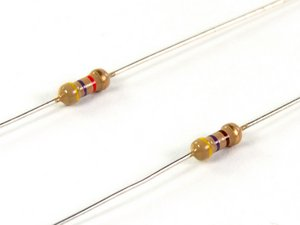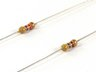주요 콘텐츠로 건너뛰기
도움말
9단계 편집 중 —

### 단계 유형:끌어서 재배열 합니다

So, what are resistors, and why do we care about them? Resistors are components used in circuitry to control the amount of current flow. The more resistance a resistor has (measured in Ohms, Ω), the less current it allows to flow.

The colored bands on a resistor are the key to determining the resistance of that particular resistor. A resistor color code chart will come in handy here.

If there are four bands on your resistor, the first band to locate is the red, gold, or silver band on one of the swollen ends; these are called tolerance bands. Since our resistors have gold bands, we know the actual resistance is within ±5% of the nominal value

The next step is to determine the nominal value of your resistor. Starting at the opposite side of the tolerance band and moving from left to right, there are three colored bands. The first two color bands correspond to a number (0-9), and the third band is the multiplier band, which corresponds to a specific power of 10.

Looking at the top resistor shown, we see yellow, violet, and red bands. Consulting a resistor color code chart shows us that those correspond to 4, 7, and 100, respectively, giving us a nominal resistance of 4,700 Ω.

Can you determine the resistance of the bottom resistor?

귀하의 기여는 오픈 소스 Creative Commons 인가 하에 허가되었습니다.